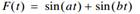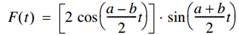### Create an Account

Already have account?

### Forgot Your Password ?

Home / Questions / Generate plots for the function in Example 8 1 with sample intervals of 0 5 sec 1 sec and ...

# Generate plots for the function in Example 8 1 with sample intervals of 0 5 sec 1 sec and 10 sec Explain the results in light of the sampling theorem Example 81 Consider the function Using a

Generate plots for the function in Example 8.1 with sample intervals of 0.5 sec, 1 sec, and 10 sec. Explain the results in light of the sampling theorem.

Example 8.1

Consider the functionUsing a trigonometric identity for the sum of two sinusoidal functions, we can rewrite F ( t ) as the following product:Jul 26 2020 View more View Less

#### Answer (Solved)Subscribe To Get Solution